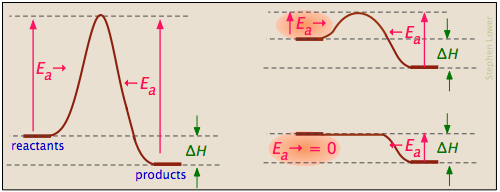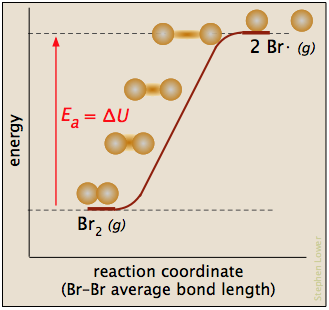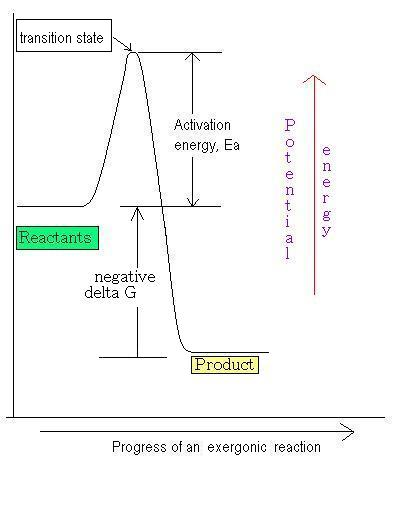# Basics of Reaction Profiles

Most reactions involving neutral molecules cannot take place at all until they have acquired the energy needed to stretch, bend, or otherwise distort one or more bonds. This critical energy is known as the activation energy of the reaction. Activation energy diagrams of the kind shown below plot the total energy input to a reaction system as it proceeds from reactants to products.In examining such diagrams, take special note of the following:

• The "reaction coordinate" plotted along the abscissa represents the changes in atomic coordinates as the system progresses from reactants to products. In the very simplest elementary reactions it might correspond to the stretching or twisting of a particular bond, and be shown to a scale. In general, however, the reaction coordinate is a rather abstract concept that cannot be tied to any single measurable and scalable quantity.
• The activated complex (also known as the transition state) represents the structure of the system as it exists at the peak of the activation energy curve. It does not correspond to an identifiable intermediate structure (which would more properly be considered the product of a separate elementary process), but rather to whatever configuration of atoms exists during the collision, which lasts for only about 0.1 picosecond.
• Activation energy diagrams always incorporate the energetics (ΔG or ΔH) of the net reaction, but it is important to understand that the latter quantities depend solely on the thermodynamics of the process which are always independent of the reaction pathway. This means that the same reaction can exhibit different activation energies if it can follow alternative pathways.
• With a few exceptions for very simple processes, activation energy diagrams are largely conceptual constructs based on the standard collision model for chemical reactions. It is unwise to read too much into them.

## Example activation energy plots

Activation energy diagrams can describe both exothermic and endothermic reactions:The activation energies of the forward reactions can be large, small, or zero (independently, of course, of the value of ΔH):Processes with zero activation energy most commonly involve the combination of oppositely-charged ions or the pairing up of electrons in free radicals, as in the dimerization of nitric oxide (which is an odd-electron molecule).In the plot below for the dissociation of bromine, Ea is the enthalpy of atomization in the following reaction:

Br2(g) → 2 Br· (g)

The reaction coordinate corresponds roughly to the stretching of the vibrationally-excited bond.The hypothetical activated complex is the last, longest "stretch". The reverse reaction, the recombination of two radicals, occurs immediately on contact.

## The origin of the activation energyIn most cases, the activation energy is supplied by thermal energy, either through intermolecular collisions or (in the case of thermal dissociation) by thermal excitation of a bond-stretching vibration to a sufficiently high quantum level.

As products are formed, the activation energy is returned in the form of vibrational energy which is quickly degraded to heat. It is worth noting, however, that other sources of activation energy are sometimes applicable:

• Absorption of light by a molecule (photoexcitation) can be a very clean and efficient, but it is not always effective. It is not sufficient that the wavelength of the light correspond to the activation energy; it must also fall within the absorption spectrum of the molecule, and (in a complex molecule) enough of it must end up in the right part of the molecule, such as in a particular bond.
• Electrochemical activation. Molecules capable of losing or gaining electrons at the surface of an electrode can undergo activation from an extra potential (known as the overvoltage) between the electrode and the solution. The electrode surface often plays an active role, so the process is also known as electrocatalysis.

A potential-energy profile is a diagram used to describe the mechanism of a reaction. This diagram is used to better illustrate the concepts of activation energy and the arrhenius equation, as well as to show the changing potential energy between the reactant and product that occur during a chemical reaction.1. The potential energy of the reactant.
2. The difference in potential energy between the reactant and the activated complex (also known as the activation energy of the forward reaction).
3. The potential energy of the activated complex (the transition state).
4. The energy difference between the product and the activated complex.
5. The difference in potential energy between the reactant and the product.
6. The potential energy of the product.

For a chemical reaction to occur, there must be a contact (collision) between the reactants. For further details about the reaction rate, see the Definition of A Reaction Rate. As the reaction proceeds, the potential energy rises to a maximum and the reactants form a cluster of atoms, called the activated complex. The highest point on the diagram is the activation energy, Ea, the energy barrier that must be overcome for a reaction to occur. Beyond the maximum, the potential energy decreases as the atoms rearrange in the cluster, until it reaches a constant state of energy. Finally, the products are formed.

The direction of a reversible reaction is determined by the transition state (also known as activated complex). There is a possibility that a collision between reactant molecules may not form products. The outcome depends on the factors mentioned in the transition state theory. If the activated complex can pass the barriers, the product forms. Otherwise, the complex falls apart and reverts to the reactants.

The graph above is an example of an elementary reaction, a single step chemical reaction with a single transition state. It does not matter whether there are one or more reactants or products. A combination of multiple elementary reactions is called a stepwise reaction. The potential energy diagram of this type of reaction involves one or more reaction intermediates. An intermediate is a species that is the product of one step of a reaction and the reactant for the next step.

The rate law of a stepwise reaction is complicated compared with that of an elementary reaction. However, there is only one slow step, the rate-determining step in the reaction. The rate-determining step controls the overall rate of the reaction, as the overall rate cannot proceed any faster than this rate-limiting step. In the potential energy profile, the rate-determining step is the reaction step with the highest energy of transition state (See: Transition State Theory). The diagram below is an example of a stepwise reaction.For the diagram above, the rate-determining step is the first reaction as the first transition state is higher than the second one. There is one intermediate in this reaction.

For the potential energy profile for a catalyzed reaction, see the articles on activation energy and Gibbs free energy. Note that catalysts increase the reaction rate by decreasing the activation energy of the reaction but do not affect the potential energy of the reactants and products.

The concepts of potential energy and Gibbs free energy are related to each other: Gibbs free energy, G0, is actually a chemical potential energy. Both quantities can be used to measure how far the reaction is from equilibrium. Here are two types of potential energy profiles based on the free energy:

1. Endergonic reaction

• The potential energy of the product is greater than that of the reactant
• Because ∆G0 > 0, the reaction absorbs energy from the environment (energy put into the system)2. Exergonic reaction

• The potential energy of the product is less than that of the reactant
• Because ∆G0 < 0, the reaction releases energy into the environment (energy out of the system)## References

1. Atkins, Peter and de Paula, Julio. (2006). Physical Chemistry for the Life Sciences. New York (NY): W.H. Freeman and Company. p. 2583-59.
2. Bruice, Paula Yurkanis. (2004). Organic Chemistry, Fourth Edition. NJ: Pearson Education, Inc. p. 135-6.

## Contributors

• Yuliani Sanjaya